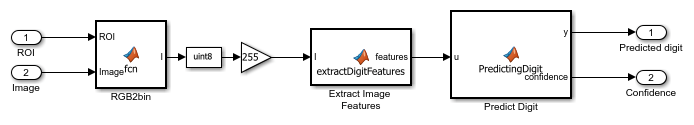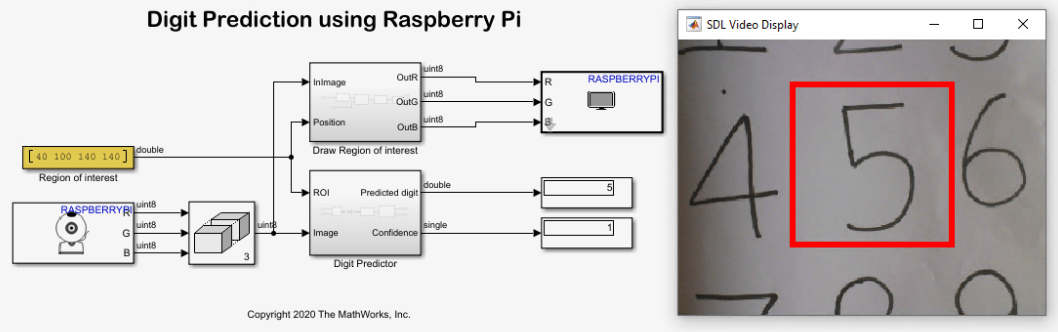# Recognize Handwritten Digits Zero to Nine Using MNIST Data Set on Raspberry Pi Hardware

This example shows how to use the Simulink® Support Package for Raspberry Pi™ Hardware to recognize images of handwritten digits from zero to nine. In this example, a web camera interfaced with a Raspberry Pi hardware board is used to capture images of the handwritten numbers. The algorithm recognizes the digits and then outputs a label for the digit along with its prediction probability.

This example uses a pretrained network, `originalMNIST.mat`, for prediction. The network has been trained using the Modified National Institute of Standards and Technology database (MNIST) data set.

The MNIST data set is commonly used data set in the field of neural networks. This data set comprises of 60,000 training and 10,000 testing grayscale images for machine learning models. Each image is 28-by-28 pixels.

### Prerequisite

For more information on how to run a Simulink model on Raspberry Pi hardware, see the Getting Started with Simulink Support Package for Raspberry Pi Hardware example.

### Required Hardware

• Raspberry Pi board

• Micro USB cable

• You can either use a USB web camera or a Raspberry Pi cameraboard. In this example, an external USB web camera is interfaced with a Raspberry Pi board.

### Hardware Setup

1. Connect the Raspberry Pi board to the host computer.

2. Connect the USB web camera to the Raspberry Pi board.

### Configure Simulink Model and Calibrate Parameters

This example uses a preconfigured Simulink model from the Simulink Support Package for Raspberry Pi Hardware. In this model, RGB images are processed using a V4L2 Video Capture block (used to capture live video) and the SDL Video Display block (used to display live video).

To open the Simulink model, run this command in the MATLAB® Command Window.

```open_system('raspberrypi_digitClassification') ```Specify a region of interest (ROI) for the camera. Specify the ROI using the Constant value parameter of the Region of interest block. The Region of interest block is a Constant block. The Region of interest block generates a bounding box around the ROI that you specify.

Specify the ROI as an N-dimensional [_x_, y, w, h] array, where x and y represent the x- and y- coordinates and w and h represent width and height and determine the size. The ROI used in this example [40 100 140 140]. You can modify these values to match your requirements.In this figure, the x and y coordinate points are 40 and 100, respectively. The value 140 represents the width of ROI from the x coordinate (w = 40 + 140 = 180) and the value 140 represents the height of ROI from the y coordinate (h = 100 + 140 = 240).

The captured image within the specified ROI is the input to the Position port on the Draw Region of interest subsystem and the ROI port on the Digit Predictor subsystem.

Configure these parameters on the V4L2 Video Capture block:

1. Enter the path and name of the video device in the Device name parameter.

Tip: You can use the Raspberry Pi Resource Monitor App to find the name of the video device connected to the Raspberry Pi board.

2. In the Image size parameter, specify the width in pixels and height in lines of the video that you want to capture.

3. Set the video format to `RGB` in the Pixel format parameter.

4. Enter the Sample time of the video device.

The Matrix Concatenate block concatenates the R, G, and B image data that it receives from the V4L2 Video Capture block to create a signal that acts as an input to the Draw Region of interest and Digit Predictor subsystems. Configure these parameters in the Matrix Concatenate block:

1. Set Number of inputs to `3` for the R, G, and B data input of the captured image.

2. Set Mode to `Multidimensional` to perform multidimensional concatenation on the R, G, and B image data input.

3. Set Concatenate dimension to `3` to specify the output dimension along which to concatenate the input array of R, G, and B image data.

Configure this parameter in the SDL Video Display block:

1. Set the Pixel format of the input video stream to `RGB`.

The Predict Digit and the Confidence Display blocks use default values.

To open the Draw Region of Interest subsystem, run this command in the MATLAB Command Window.

```open_system('raspberrypi_digitClassification/Draw Region of interest') ```The Draw Region of Interest subsystem draws the ROI starting from (40, 100) to (180, 240) pixels. To draw the ROI, this image is converted to single format and then converted back to RGB.

To open the Digit Predictor subsystem, run this command in the MATLAB Command Window.

```open_system('raspberrypi_digitClassification/Digit Predictor') ```In the Digit Predictor subsystem, the RGB2bin block converts the image into its binary equivalent and then extracts the ROI from the input image. The block complements and resizes the image to 28-by-28 pixels. It passes the resized image to the Extract Image Features block to extract the histogram of oriented gradients (HOG) features. The extracted features go to the Predict Digit block. The Predict Digit block loads the compact trained model, `originalMNIST.mat`, to predict the digits from the extracted features. For more information on how the `originalMNIST.mat` file is trained, see the Digit Classification Using HOG Features on MNIST Database (Simulink Support Package for Android Devices) example. The Predict Digit block displays the predicted digit and the Confidence (0-1) block displays its probability of prediction.

The web camera connected to the Raspberry Pi hardware board captures the image of the digit that is to be recognized. The outputs such as the ROI marked around the input image, predicted digit, and confidence value are displayed on the SDL Video Display, Predicted Digit Display block, and Confidence (0-1) Display block, respectively.

1. Draw digits 0 to 9 on a white board or a white sheet of paper. In this example, the support package recognizes the digit `5`.

2. Capture the digit `5` using the web camera. Ensure that the digit is enclosed inside the ROI.

3. On the Hardware tab of the Simulink model, in the Mode section, select Run on board and then click Monitor & Tune to run the Simulink model on the Raspberry Pi board.

4. Observe the SDL Video Display, Predicted Digit Display, and Confidence (0-1) Display blocks. The Predicted Digit Display block displays the output as `5`, and the Confidence (0-1) Display block displays the output as `1`, indicating an accurate recognition of the digit `5`.3. Click Stop to stop the Simulink model simulation.

### Other Things to Try

Try recognizing all the digits from 0 to 9.